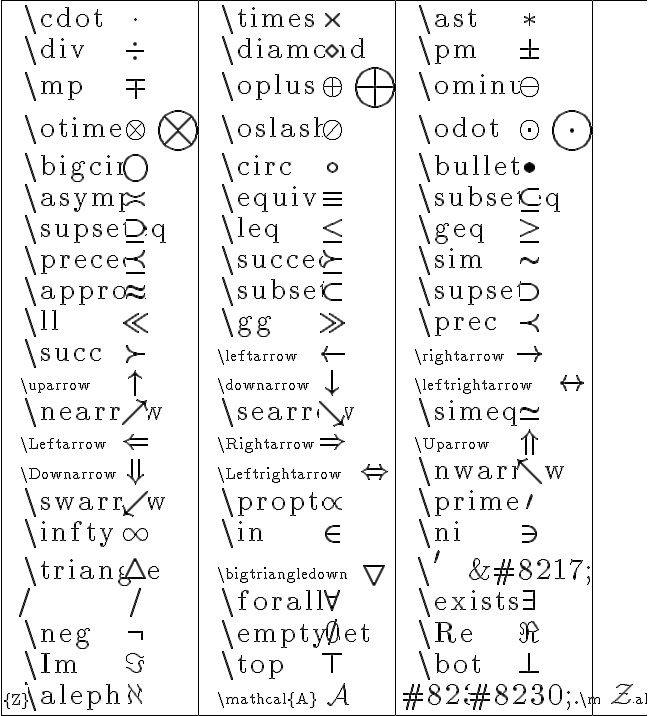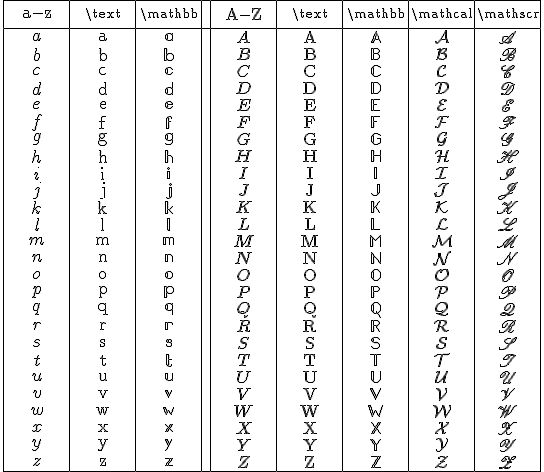## Latex rendering update

Using equation on this blog asks that you put $sign at the beginning and$sign at the end to substitute in bracket [ ]-tex and / tex

Here is the site language that will help blog developers with their latex language and give them an alternative from having to shift over to word press.

Test

$\odot$ $\oplus$ $\pi$ $\omega$

$\LARGE U=\frac{-GMm}r=\frac{-GMh}{rc^2}{vo}$

$\large hv=hv_o[{1-}\frac{GM}{rc^2}] \hspace9 v=v_o[{1-}\frac{GM}{rc^2}] \hspace9 \frac{\bigtriangledown v} {v_o}={-}\frac{GM}{rc^2}$

***Click on Image for a larger sizeClick on Image for a larger sizeClick on Image for a larger size

***

This entry was posted in Blog Developers, latex rendering and tagged , . Bookmark the permalink.

### 8 Responses to Latex rendering update

1.Plato says:

Testing comment section$\LARGE U=\frac{-GMm}r=\frac{-GMh}{rc^2}{vo}$

2.PlatoHagel says:

Testing comment section$\\LARGE U=\\frac{-GMm}r=\\frac{-GMh}{rc^2}{vo}$

3.watchmath says:

For a post with lots of math symbols you probably need to remove the border on the image by fiddling around with the css.

4.Plato says:

Hi Watchmath,Thanks for taking the time. Under a blog format what would I look for? I posted it under the script you had given so I am not sure how this is translated through to the blog format? Any pointers or ideas here?Best,

5.PlatoHagel says:

Hi Watchmath,Thanks for taking the time. Under a blog format what would I look for? I posted it under the script you had given so I am not sure how this is translated through to the blog format? Any pointers or ideas here?Best,

6.Leon says:

$2+6x*sqrt(9)$

7.Unknown says:

$2+6x*sqrt(9)$

8.Napoleon says:

Thanks for the post, I was searching for the \mathcal{X} symbol's command for a long time.